Courses

# Test: Torsion Level - 2

## 10 Questions MCQ Test Strength of Materials (SOM) | Test: Torsion Level - 2

Description
This mock test of Test: Torsion Level - 2 for Mechanical Engineering helps you for every Mechanical Engineering entrance exam. This contains 10 Multiple Choice Questions for Mechanical Engineering Test: Torsion Level - 2 (mcq) to study with solutions a complete question bank. The solved questions answers in this Test: Torsion Level - 2 quiz give you a good mix of easy questions and tough questions. Mechanical Engineering students definitely take this Test: Torsion Level - 2 exercise for a better result in the exam. You can find other Test: Torsion Level - 2 extra questions, long questions & short questions for Mechanical Engineering on EduRev as well by searching above.
QUESTION: 1

### A shaft of 9.0m length is subjected to a torque of 300.0kN-m at a distance of 3.0m from one end. The maximum torque in the shaft is

Solution: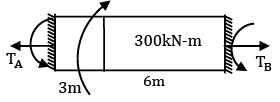Short-cut method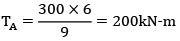TB =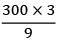= 100kN­m

∴ Maximum torque = 200kN­m

QUESTION: 2

### The minimum diameter of solid steel shaft that will not twist through more than 3° (degrees) in a 6 metre length. When subjected to a torque of 14 kN-m with modulus of rigidity G = 83 GN/m2, is equal to

Solution: Given, θ = 3° = 3 ×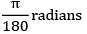I = 6m; T = 14 kN − m; G = 83GN/m2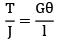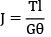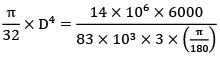= 118mm

QUESTION: 3

### A shaft of J polar moment of inertia and C modulus of rigidity is fixed at one end and subjected to torque T at the free end and the same torque at mid length in opposite direction as shown in figure then the difference in the twist between the free end and the midpoint is equal to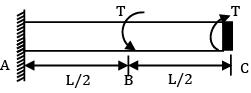Solution: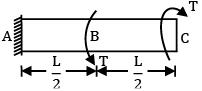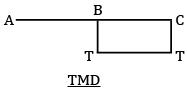We can divide this shaft into two parts AB and BC

θB = 0

Since there is no torque in AB portion

θC − θB =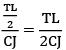QUESTION: 4

If the diameter of a shaft subjected to torque alone is doubled, then horse power P can be increase to

Solution: Given: P =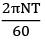P ∝ T But, T ∝ d3

P ∝ d3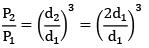P2 = 8P1

QUESTION: 5

The ratio of torque carrying capacity of a solid shaft to that of a hollow shaft is given Where K =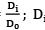= Inside diameter of hollow shaft and Do = Outside diameter of hollow shaft. Shaft material is the same.

Solution: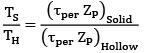ZP = Polar section modulus Since the material is same, τper will be the same for hollow and solid shaft.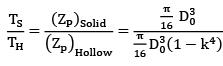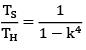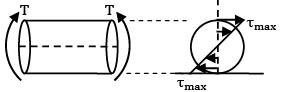QUESTION: 6

Shafts of the same material and same lengths are subjected to the same torque. If the first shaft is a solid circular section (D), and the second shaft is of hollow circular section whose internal diameter is 2⁄3 of the outside diameter (Do ), and the max. shear stress developed in each is same D ⁄ D0 = ?

Solution: Maximum shear stress is same τsolid = τhollow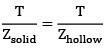Zhollow = Zsolid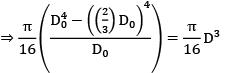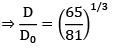QUESTION: 7

The ratio of the strength of a solid shaft and hollow shaft of the same external diameter and internal diameter half of the external diameter is

Solution: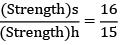= 1.07

=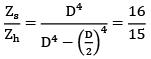= 1.07

QUESTION: 8

Two shafts A and B are of same length and subjected to same torque T. If the diameter of shaft B is twice that of shaft A, the ratio of shear stresses developed in shafts A and B is

Solution: Given: We know,

τ =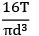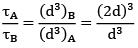= 23 = 8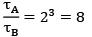QUESTION: 9

Two shafts A and B made of the same material transmit 100kW each. Shaft A turns at 250rpm while B at 300rpm. Which one has greater diameter?

Solution: P = 2πNT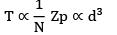↑ dia: ↓ N = ′A′

QUESTION: 10

Find the maximum shear stress induced in a solid circular shaft of diameter 200mm when the shaft transmits 190 kW power at 200 rpm.

Solution: Power, P =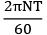190 × 103 =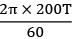τ = 9.071kN/m

τ =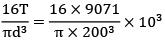τ = 5.78N/mm2